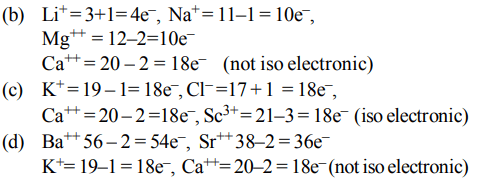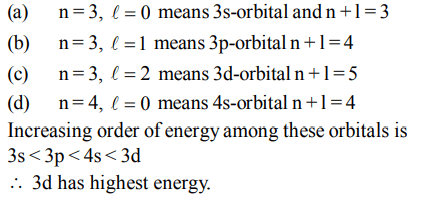## Atomic Structure Questions and Answers Part-16

1. Consider the ground state of Cr atom (X = 24). The number of electrons with the azimuthal quantum numbers, l = 1 and 2 are, respectively
a) 16 and 4
b) 12 and 5
c) 12 and 4
d) 16 and 5

Explanation: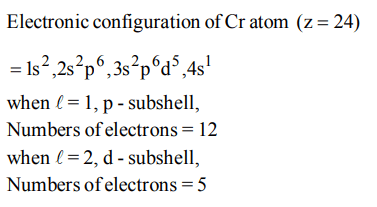2. The wavelength of the radiation emitted, when in a hydrogen atom electron falls from infinity to stationary state 1, would be$\left(Rydberg constant = 1.097×10^{7}m^{-1}\right)$
a) 406 nm
b) 192 nm
c) 91 nm
d) $9.1×10^{-8}nm$

Explanation: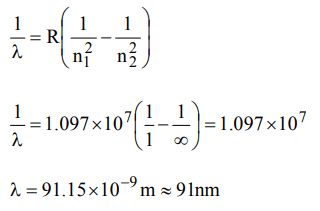3. Which one of the following sets of ions represents the collection of isoelectronic species?
a) $K^{+},Cl^{-},Mg^{2+},Sc^{3+}$
b) $Na^{+},Ca^{2+}, Sc^{3+},F^{-}$
c) $K^{+},Ca^{2+}, Sc^{3+},Cl^{-}$
d) $Na^{+},Mg^{2+}, AI^{3+},Cl^{-}$

Explanation: $_{19}K^+$, $_{20}Ca^{2+}$, $_{21}Sc^{3+}$, $_{17}Cl^-$ each contains 18 electrons

4. Of the following outer electronic configurations of atoms, the highest oxidation state is achieved by which one of them ?
a) $\left(n-1\right)d^{3}ns^{2}$
b) $\left(n-1\right)d^{5}ns^{1}$
c) $\left(n-1\right)d^{8}ns^{2}$
d) $\left(n-1\right)d^{5}ns^{2}$

Explanation: (n–1)d5ns2 attains the maximum O.S. of + 7

5. In a multi-electron atom, which of the following orbitals described by the three quantum members will have the same energy in the absence of magnetic and electric fields ?
(A) n = 1, l = 0, m = 0
(B) n = 2, l = 0, m = 0
(C) n = 2, l = 1, m = 1
(D) n = 3, l = 2, m = 1
(E) n = 3, l = 2, m = 0
a) (D) and (E)
b) (C) and (D)
c) (B) and (C)
d) (A) and (B)

Explanation: The energy of an orbital is given by (n + l) in (d) and (c). (n + l) value is (3 + 2) = 5 hence they will have same energy.

6. Of the following sets which one does NOT contain isoelectronic species?
a) $BO_3^{3-},CO_3^{2-},NO_3^-$
b) $SO_3^{2-},CO_3^{2-},NO_3^-$
c) $CN^{-},N_{2},C_2^{2-}$
d) $PO_3^{4-},SO_4^{2-},CIO_4^-$

Explanation: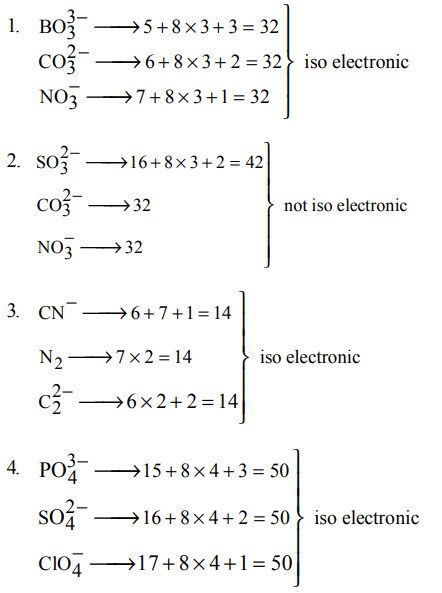7. According to Bohr's theory, the angular momentum of an electron in 5th orbit is
a) $10 h/\pi$
b) $2.5 h/\pi$
c) $25 h/\pi$
d) $1.0 h/\pi$

Explanation:8. Uncertainty in the position of an electron $\left(mass=9.1 ×10^{-31}kg\right)$     moving with a velocity $300ms^{-1}$ , accurate upto 0.001% will be $\left(h = 6.63 × 10^{-34}Js\right)$
a) $1.92 × 10^{-2}m$
b) $3.84 × 10^{-2}m$
c) $19.2 × 10^{-2}m$
d) $5.76× 10^{-2}m$

Explanation:9. Which one of the following sets of ions represents a collection of isoelectronic species?
a) $N^{3-},O^{2-},F^{-},S^{2-}$
b) $Li^{+},Na^{+},Mg^{2+},Ca^{2+}$
c) $K^{+},Cl^{-},Ca^{2+},SC^{3+}$
d) $Ba^{2+},Sr^{2+},K^{+},Ca^{2+}$